Test: SAT Math

 1 On the coordinate plane,,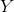, and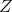are the points with coordinates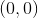,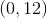, and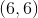, respectively. Lines,, andare the perpendicular bisectors of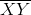,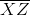, and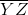, respectively.andintersect at a point;andintersect at a point;andintersect at a point. Which of these statements is true of,, and?andare the same point;is a different point., andare distinct and are the vertices of an equilateral triangle., andare the same point., andare distinct and collinear., andare distinct and are the vertices of a triangle similar to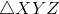.

1/2 questions

0%

Access results and powerful study features!

Take 15 seconds to create an account.